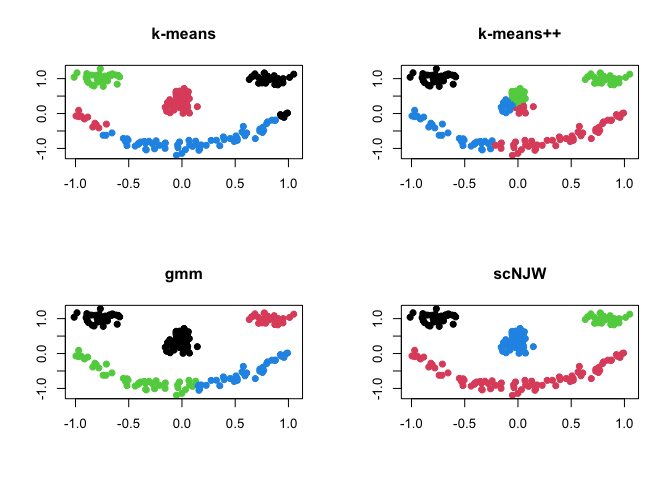# Tools for Cluster AnalysisT4cluster is an R package designed as a computational toolkit with comprehensive coverage in relevant topics around the study of cluster analysis. It contains several classes of algorithms for

• Clustering with Vector-Valued Data
• Clustering with Functional Data
• Clustering on the Unit Hypersphere
• Subspace Clustering
• Measures : Compare Two Clusterings
• Learning with Multiple Clusterings

## Installation

You can install the released version of T4cluster from CRAN with:

``install.packages("T4cluster")``

And the development version from GitHub with:

``````# install.packages("devtools")
devtools::install_github("kisungyou/T4cluster")``````

## Minimal Example : Clustering SMILEY Data

T4cluster offers a variety of clustering algorithms in common interface. In this example, we show a basic pipeline with `smiley` dataset, which can be generated as follows;

``````# load the library
library(T4cluster)

# generate the data
smiley = T4cluster::genSMILEY(n=200)
data   = smiley\$data
label  = smiley\$label

# visualize
plot(data, pch=19, col=label, xlab="", ylab="", main="SMILEY Data")``````where each component of the face is considered as one cluster - the data has 4 clusters. Here, we compare 4 different methods; (1) k-means (`kmeans`), (2) k-means++ (`kmeanspp`), (3) gaussian mixture model (`gmm`), and (4) spectral clustering with normalized cuts (`scNJW`).

``````# run algorithms
run1 = T4cluster::kmeans(data, k=4)
run2 = T4cluster::kmeanspp(data, k=4)
run3 = T4cluster::gmm(data, k=4)
run4 = T4cluster::scNJW(data, k=4, sigma = 0.1)

# visualize
par(mfrow=c(2,2))
plot(data, pch=19, xlab="", ylab="", col=run1\$cluster, main="k-means")
plot(data, pch=19, xlab="", ylab="", col=run2\$cluster, main="k-means++")
plot(data, pch=19, xlab="", ylab="", col=run3\$cluster, main="gmm")
plot(data, pch=19, xlab="", ylab="", col=run4\$cluster, main="scNJW")``````